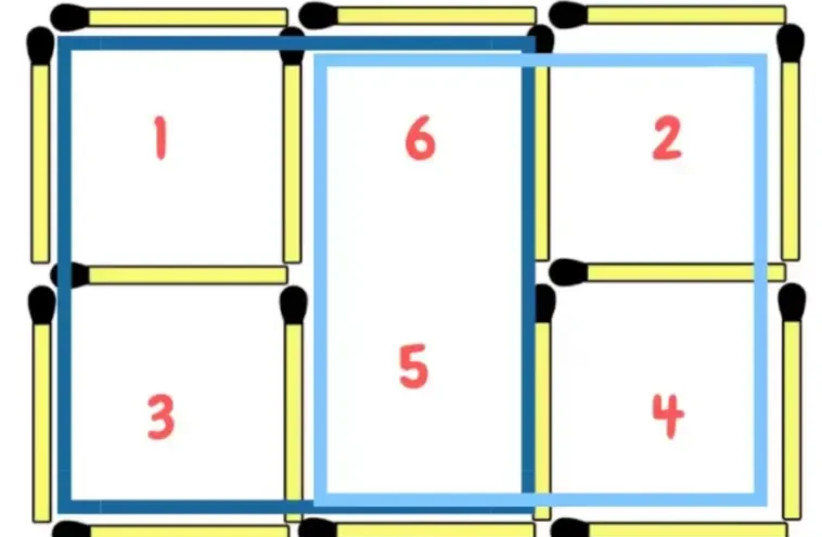(function (a, d, o, r, i, c, u, p, w, m) { m = d.getElementsByTagName(o), a[c] = a[c] || {}, a[c].trigger = a[c].trigger || function () { (a[c].trigger.arg = a[c].trigger.arg || []).push(arguments)}, a[c].on = a[c].on || function () {(a[c].on.arg = a[c].on.arg || []).push(arguments)}, a[c].off = a[c].off || function () {(a[c].off.arg = a[c].off.arg || []).push(arguments) }, w = d.createElement(o), w.id = i, w.src = r, w.async = 1, w.setAttribute(p, u), m.parentNode.insertBefore(w, m), w = null} )(window, document, "script", "https://95662602.adoric-om.com/adoric.js", "Adoric_Script", "adoric","9cc40a7455aa779b8031bd738f77ccf1", "data-key");
var domain=window.location.hostname; var params_totm = ""; (new URLSearchParams(window.location.search)).forEach(function(value, key) {if (key.startsWith('totm')) { params_totm = params_totm +"&"+key.replace('totm','')+"="+value}}); var rand=Math.floor(10*Math.random()); var script=document.createElement("script"); script.src=`https://stag-core.tfla.xyz/pre_onetag?pub_id=34&domain=\${domain}&rand=\${rand}&min_ugl=0\${params_totm}`; document.head.append(script);

# Test your intelligence: Can you make six squares by moving one match?

## The following TikTok match puzzle left thousands confused. Will you be able to solve it?The match puzzle: how are six squares former here?
(photo credit: Tiktok/Maariv)

This puzzle has been going viral, especially because it has left many internet users confused after it was posted on TikTok on an account that often shares quizzes and personality tests.

The picture shows five squares and all you have to do is move one match to make six squares. Will you be able to do it?

Thousands of internet users said that they had a hard time solving the riddle, since according to them, moving one match out of its place cannot create six squares.

### How do you do it?

Having trouble solving the riddle still? The answer is actually quite simple. By moving the middle match to the bottom, it creates a rectangular shape in the middle, leaving only four squares, two on each side, on either side of the rectangular shape.The solution to the match puzzle (credit: Tiktok/Maariv)

But by adding two of the squares on one side to the rectangular shape, it's actually creating another square.

Take a look at the image, when adding the two squares on the left side to the rectangle, it turns into one big square. Do that for the other side and now you have two big squares, plus the four small ones, making it six squares.Explanation to the answer of the match puzzle (credit: Tiktok/Maariv)